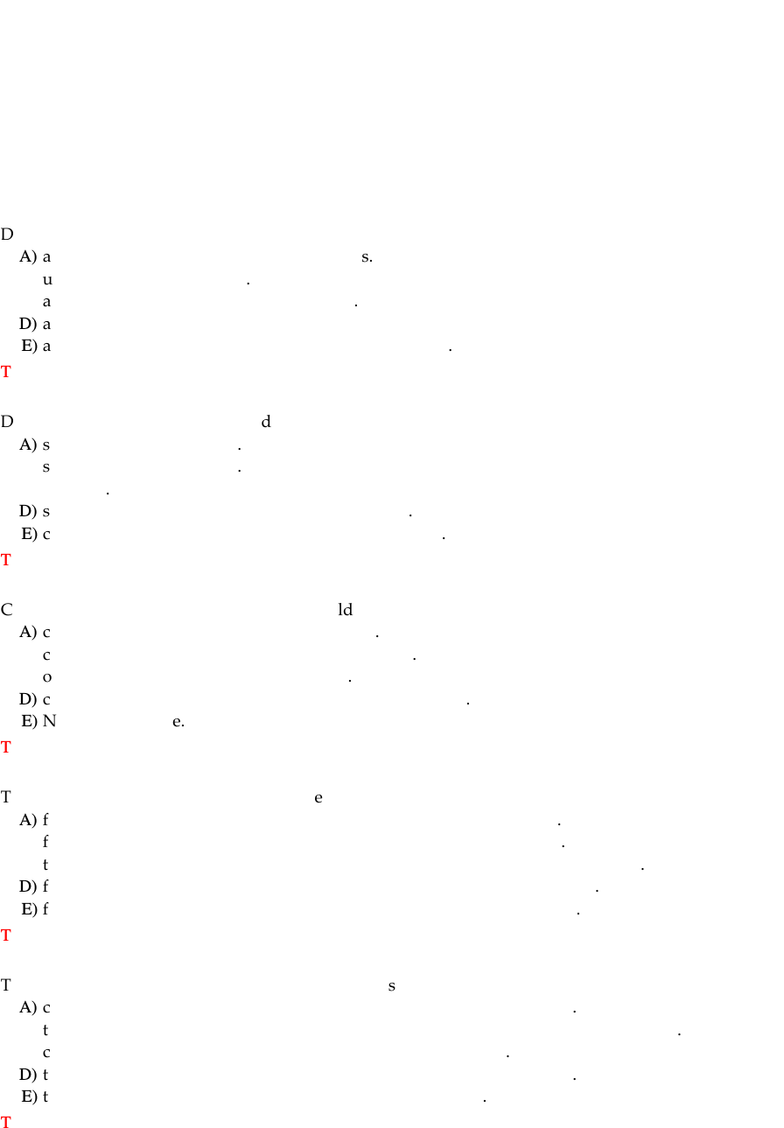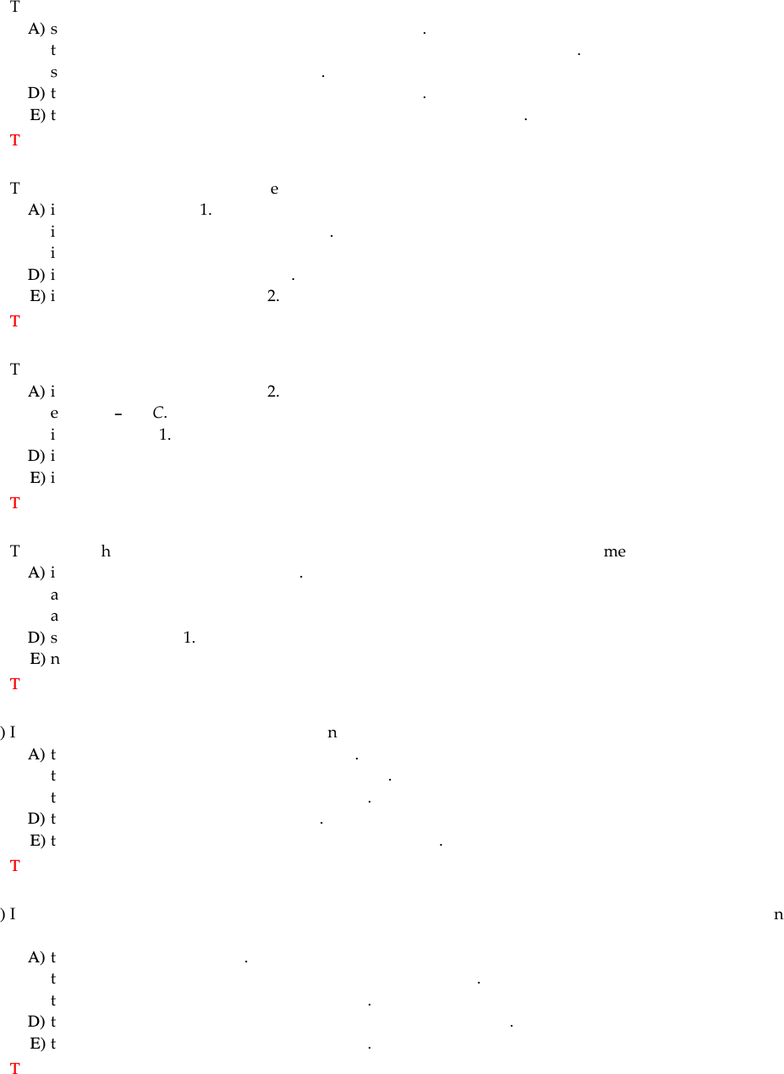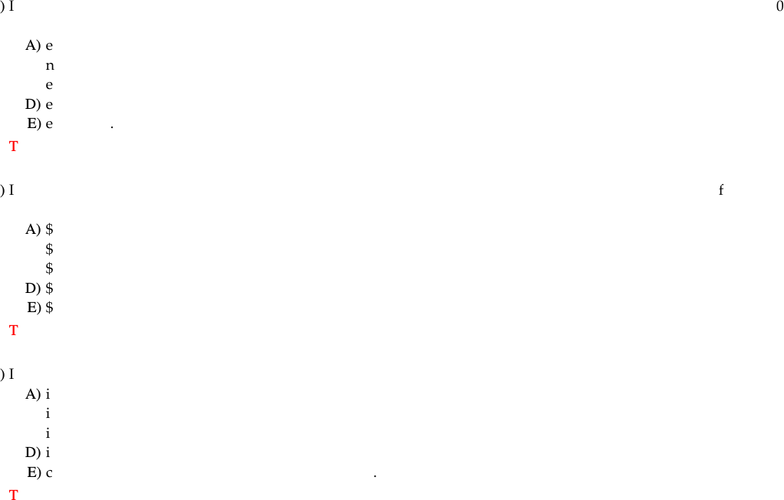Study Guides (380,000)
CA (150,000)
Ryerson (10,000)
ECN (400)
ECN 204 (100)
Final

# ECN 204 Lecture Notes - Lecture 4: Disposable And Discretionary Income, Grain Elevator, European Route E40Exam

Department
Economics
Course Code
ECN 204
Professor
eric nam
Study Guide
Final

This preview shows pages 1-3. to view the full 33 pages of the document.University of Lethbridge
Department of Economics
ECON 1012
Introduction to Macroeconomics
Instructor: Michael G. Lanyi
CH 27 Expenditure Multipliers
1)
Disposable income is
A)
aggregate income minus transfer payments.
B)
used for consumption only.
C)
D)
aggregate income minus taxes.
E)
aggregate income minus taxes plus transfer payments.
Topic:
Fixed Prices and Expenditure Plans
2)
Dissaving occurs when a household
A)
saves more than it spends.
B)
spends more than it saves.
C)
borrows.
D)
spends less than it receives in disposable income.
E)
consumes more than it receives in disposable income.
Topic:
Fixed Prices and Expenditure Plans
3)
Complete the following sentence. A household
A)
consumes or saves out of disposable income.
B)
consumes or pays taxes out of disposable income.
C)
only
consumes out of disposable income.
D)
consumes, saves, or pays taxes out of disposable income.
E)
None of the above.
Topic:
Fixed Prices and Expenditure Plans
4)
The marginal propensity to consume is the
A)
fraction of the last dollar of disposable income received that is saved.
B)
fraction of the first dollar of disposable income received that is saved.
C)
total amount of consumption divided by the total amount of disposable income.
D)
fraction of the first dollar of disposable income received that is consumed.
E)
fraction of a change in disposable income that is spent on consumption.
Topic:
Fixed Prices and Expenditure Plans
5)
The marginal propensity to consume is calculated as
A)
consumption expenditure divided by the change in disposable income.
B)
the change in consumption expenditure divided by the change in disposable income.
C)
consumption expenditure divided by total disposable income.
D)
the change in consumption expenditure divided by disposable income.
E)
the change in consumption expenditure divided by saving.
Topic:
Fixed Prices and Expenditure Plans
1

Only pages 1-3 are available for preview. Some parts have been intentionally blurred.6)
The marginal propensity to save is calculated as
A)
saving divided by the change in disposable income.
B)
the change in saving divided by the change in consumption expenditure.
C)
saving divided by disposable income.
D)
the change in saving divided by disposable income.
E)
the change in saving divided by the change in disposable income.
Topic:
Fixed Prices and Expenditure Plans
7)
The marginal propensity to consume
A)
is between zero and 1.
B)
is greater than 1 if dissaving is present.
C)
is between 1/2 and 1.
D)
is negative if dissaving is present.
E)
is greater than 1 but less than 2.
Topic:
Fixed Prices and Expenditure Plans
8)
The marginal propensity to save
A)
is greater than 1 but less than 2.
B)
equals 1
MPC
.
C)
is greater than 1.
D)
is between zero and 1/2.
E)
is negative.
Topic:
Fixed Prices and Expenditure Plans
9)
The sum of th
e marginal propensity to save and the marginal propensity to consume
A)
is greater than zero but less than 1.
B)
always equals 1.
C)
always equals 0.
D)
sometimes
equals 1.
E)
never equals 1.
Topic:
Fixed Prices and Expenditure Plans
10)
If the marginal propensity to save is 0.2, then
A)
the marginal propensity to consume is 0.8.
B)
the marginal propensity to consume is also 0.2.
C)
the slope of the consumption function is 0.2.
D)
the slope of the saving function is 0.8.
E)
the marginal propensity to consume is larger than 0.8.
Topic:
Fixed Prices and Expenditure Plans
11)
If a household's disposable income increases from \$12,000 to \$22,000 and at the same time its consumption
expenditure increases from \$4,000 to \$9,000, then
A)
the household is dissaving.
B)
the marginal propensity to save over this range is negative.
C)
the slope of the consumption function is 0.6.
D)
the marginal propensity to consume over this range is negative.
E)
the slope of the consumption function is 0.5.
Topic:
Fixed Prices and Expenditure Plans
2

Only pages 1-3 are available for preview. Some parts have been intentionally blurred.12)
If consumption expenditure for a household increases from \$300 to \$500 when disposable income increases from \$200
to \$500, the marginal propensity to consume is
A)
equal to 1.33.
B)
negative.
C)
equal to 0.67.
D)
equal to 0.75.
E)
equal to 1.
Topic:
Fixed Prices and Expenditure Plans
13)
If the marginal propensity to consume is 0.85, what change in consumption expenditure would you expect if
disposable income increases by \$200 million?
A)
\$170 million
B)
\$18 million
C)
\$1,800 million
D)
\$20 million
E)
\$180 million
Topic:
Fixed Prices and Expenditure Plans
14)
If consumption is \$8,000 when disposable income is \$10,000, the marginal propensity to consume
A)
is 1.25.
B)
is 0.80.
C)
is 0.75.
D)
is 0.50.
E)
cannot be determined from the information given.
Topic:
Fixed Prices and Expenditure Plans
3